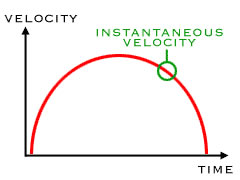# Difference between Velocity and Instantaneous Velocity

##### Key Difference: Velocity refers to the rate of change of distance with respect to time. It is a vector quantity, which means that it has both - a direction and a magnitude. Instantaneous velocity can be described as the rate at which an object is moving at a specific moment.Velocity is defined as the rate of change of distance with respect to time. It is a vector quantity, which means that it has both - a direction and a magnitude. It can also be mentioned as a speed with a direction. Velocity keeps the track of direction, and thus direction plays a vital role in the calculation of the velocity. One needs to specify the direction in order to provide the complete information regarding the velocity of an object. The units of velocity are m/sec, cm/sec, ft/sec, km/h, miles/hr, etc.  Velocity can be broadly divided into two groups - Average Velocity and Instantaneous Velocity. The average velocity depends only on the initial and final points of the travel. On the other hand, instantaneous velocity provides the information pertaining to a single point.Instantaneous velocity can be found by determining the slope of position vs time graph at a particular time for which the calculation is required. Instantaneous velocity is different from the average velocity. However, If a car travels from A to B, then the instantaneous velocity at A can also be decribed as the average velocity between A and B as B approaches A.

Comparison between Velocity and Instantaneous Velocity:

 Velocity Instantaneous Velocity Definition Velocity refers to the rate of change of distance with respect to time. It is a vector quantity, which means that it has both - a direction and a magnitude. Instantaneous velocity can be described as the rate at which an object is moving at a specific moment. Formula Velocity = displacement/time Instantaneous velocity = LimΔT → 0 ΔS / ΔT = dS / dT Units m/sec, cm/sec, ft/sec, km/h, miles/hr, etc. m/sec, cm/sec, ft/sec, km/h, miles/hr, etc. Example If a car comes back to its initial position, then the displacement is zero. Therefore, this motion results in zero velocity. The speedometer on a car's dashboard reads instantaneous speed. Purpose Velocity tells you that how fast and in what direction. Instantaneous velocity represents the velocity measured over an infinitesimally small time interval.

Image Courtesy: physicsclassroom.com, physics4kids.com